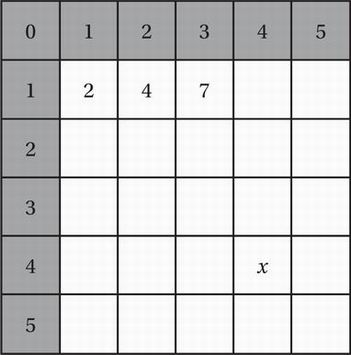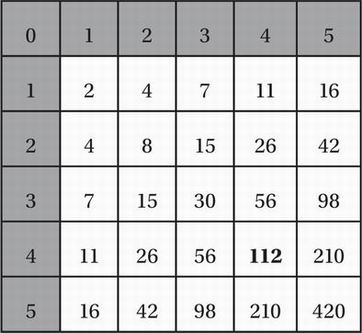# SAT Math Multiple Choice Question 716: Answer and Explanation

### Test Information

Question: 716

11.With the exception of the shaded squares in the first row and first column, every square in the table above is to be filled in with a number equal to the sum of the number directly above it and the number directly to its left. For instance, the number 7 in the second row is the sum of 3 in the square above it and 4 in the square directly to its left. What is the value of x?

• A. 16
• B. 84
• C. 96
• D. 112